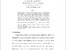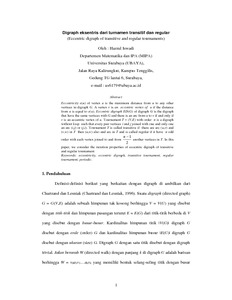# Digraph eksentris dari turnamen transitif dan regular

Iswadi, Hazrul (2003) Digraph eksentris dari turnamen transitif dan regular. Matematika dan Ilmu Pengetahuan Alam, 8 (2). pp. 63-67. ISSN 0852-4556Preview PDF hazrul_Digraph eksentris dari turnamen transitif dan regular_2003.pdf Download (65kB) | Preview

## Abstract

Eccentricity e(u) of vertex u is the maximum distance from u to any other vertices in digraph G. A vertex v is an eccentric vertex of u if the distance from u is equal to e(u). Eccentric digraph ED(G) of digraph G is the digraph that have the same vertices with G and there is an arc from u to v if and only if v is an eccentric vertex of u. Tournament T = (V,E) with order n is a digraph without loop such that every pair vertices i and j joined with one and only one an arc (i,j) or (j,i). Tournament T is called transitive if there are arc (u,v) and (v,w) in T then (u,w) also and arc in T and is called regular if it have n odd order with each vertex joined to and from (n-1)/2 another vertices in T. In this paper, we consider the iteration properties of eccentric digraph of transitive and regular tournament

Item Type: Article eksentrisitas, digraf eksentrik Q Science > QA Mathematics Academic Department > Department of Mathematics and Natural Science Hazrul Iswadi 6179 15 Mar 2012 06:44 20 Mar 2012 01:33 http://repository.ubaya.ac.id/id/eprint/196View Item